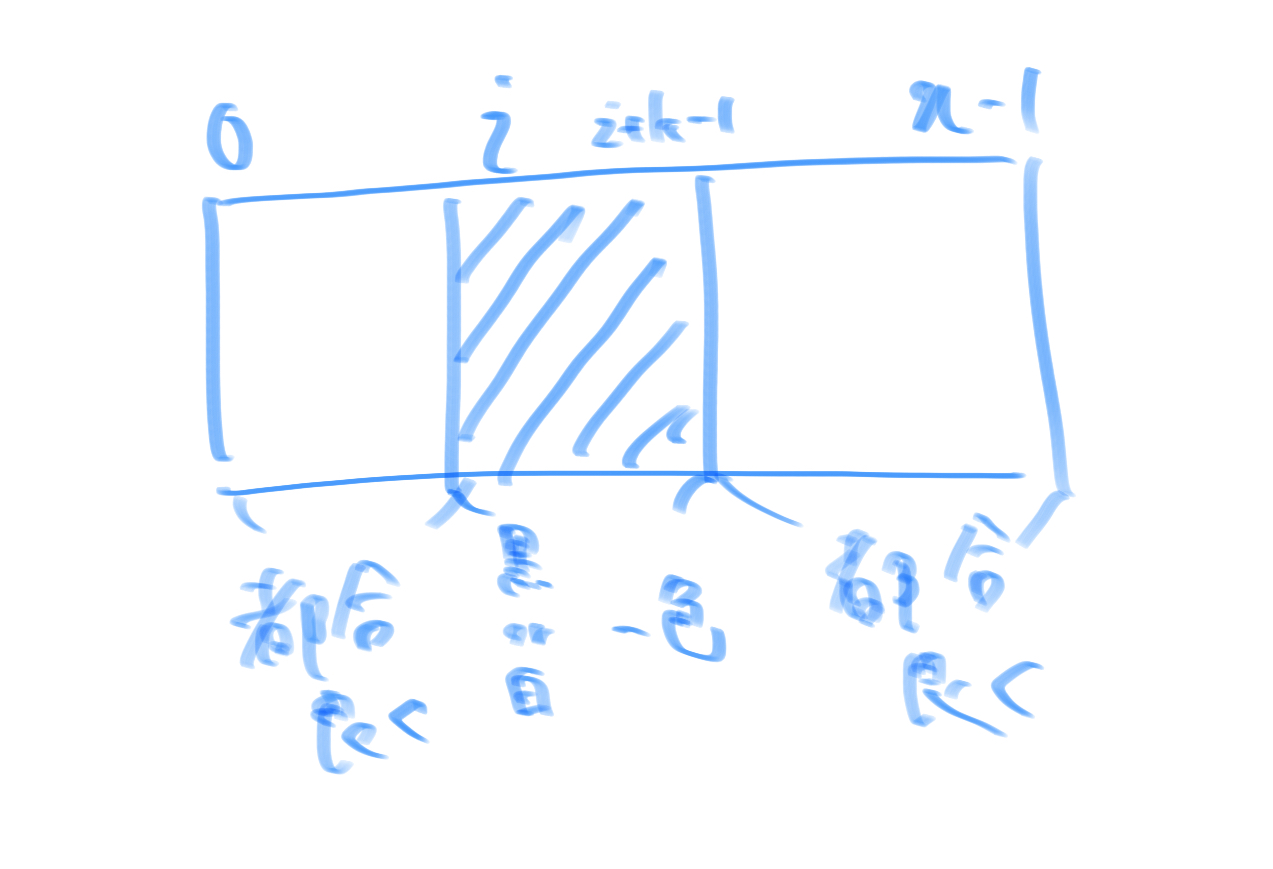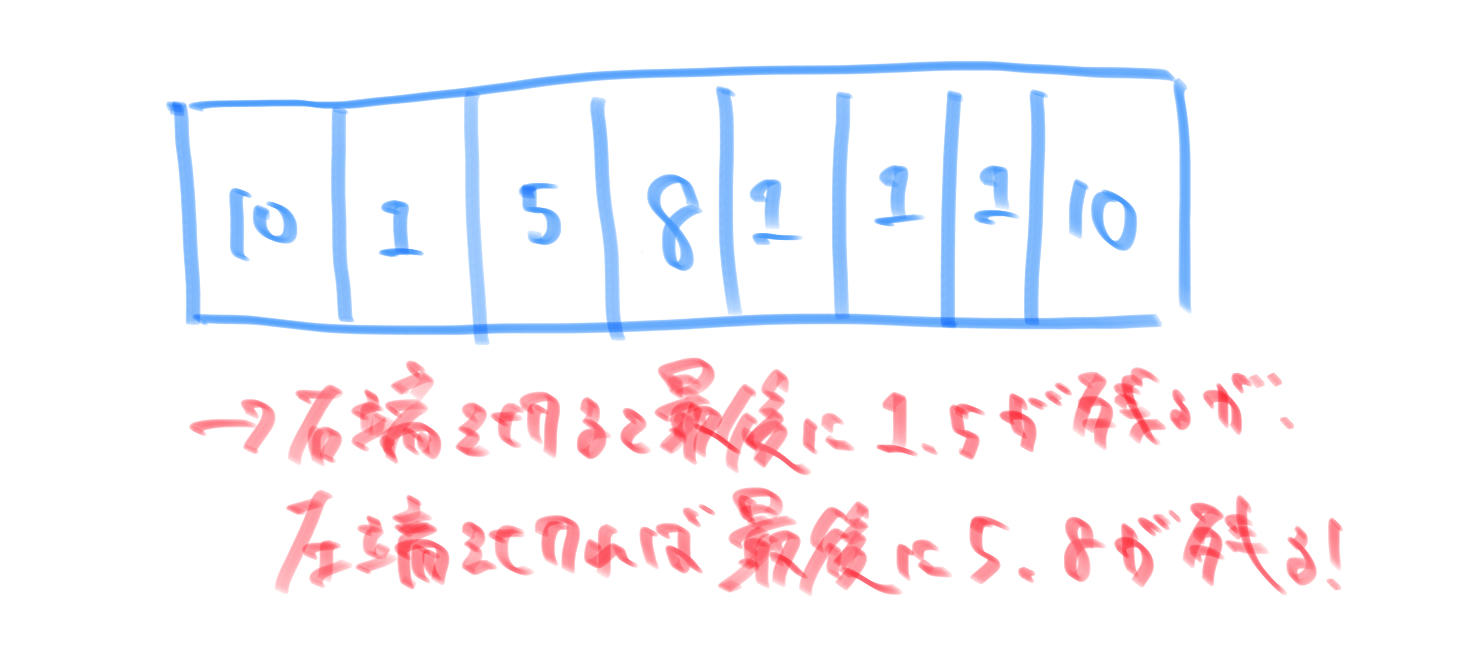[PYTHON] AtCoder devotion memo (11/12)

It is a memo of devotion in AtCoder. I'm sorry that there is a mixture of tones and tones.

AGC008B - Contiguous Repainting

diff:1821 Result: 20 minutes for consideration, 10 minutes for basic implementation, ∞ minutes for bug fixing → lambda expression of Accumulate function was wrong

Submission: https://atcoder.jp/contests/agc008/submissions/18058051

Consideration

First, consider ** painting as much as you can conveniently ** (black for \$ a \ _i \ geqq 0 \$, white for \$ a \ _i \ <0 \$). At this time, it seemed that the humor would be clear even if I applied it properly, so I thought about ** painting in order from the edge **. At this time, you can conveniently paint all but the \$ k \$ squares at the edges, and all the \$ k \$ squares at the edges can be painted black or white. Therefore, I thought that it would be better to paint the \$ k \$ squares on the left end conveniently and the \$ k \$ squares on the right end conveniently, but as in sample 2, the \$ between ** ** may be optimal if only k \$ cells cannot be painted conveniently. Therefore, I thought that if these were ** generalized **, the continuous \$ k \$ cells could be painted in one color with white or black, and the other parts could be painted in a convenient way. At this time, I solved it by showing only sufficiency, but if I calm down, by overwriting at the end, \$ k \$ cells that are continuous in one color will appear, so ** I can also show the necessity. **. Therefore, if you just implement this using the cumulative sum, it will be as follows if you write it in a diagram.At this point, I just implemented it, but I made a mistake ** because I used a lambda expression for the function passed to the ** accumulate function. I'd like to ** pass preprocessed iterables to the accumulate function **.

Also, it seems to be a typical idea to reverse the operation ** in the problem of overwriting. That's true, and overwriting is a destructive operation, whereas it's convenient because it can be made non-destructive by reversing it **. I feel that this is similar to the fact that it is convenient to regard it as the reverse order when cutting edges in a Union Find-like problem.

Reflection

** I made a mistake because I was trying to keep the code concise **, as in the previous issue ** I don't skip the implementation ** (learn ...), I'm sorry for the thoughts ...

DDPC2020 Qualifying D --Digit Sum Replace

diff:1650 Result: Discussion: About 25 minutes, Implementation: None, AC Submission: https://atcoder.jp/contests/ddcc2020-qual/submissions/18059365

Consideration

At first, I experimented and wondered what would happen to the combination of numbers that would be the minimum, but I thought that it would be difficult to simulate honestly because the number is large.

Therefore, when focusing on the operations, I noticed that they can be divided into ** (1) operations that reduce the number of digits and (2) operations that do not reduce the number of digits **. At this time, I noticed that in the case of (1), the number of digits is only reduced and the sum of numbers does not change **, and in the case of (2), the number of digits is not reduced but the sum of numbers is uniformly reduced by 9.

Here, in other words, the final condition should be one digit and the sum of numbers should be 9 or less so as to match the operations of ① and ②. Also, I noticed that the operations ** ① and ② are independent of each other and do not interfere with each other **, so the number of times is ** uniquely determined from the original number of digits and the original sum of numbers **. Here, the former can be easily obtained by (the number of original digits) -1, but the latter is not (the quotient of the original sum of numbers divided by 9), but when the remainder after dividing by 9 is 0, it is from that value. It is necessary to -1. Therefore, the maximum number of rounds is the sum of the number of operations (1) and (2).

✳︎ Digit sum… Total number of each digit

Reflection

It took me a while to notice the gag, and for a moment when I noticed it, this type is difficult, but ** it's good to pay attention to the amount of change in operation **?

AGC002C - Knot Puzzle

diff:1564 Result: Discussion + Implementation: 15 minutes, 15 minutes to fix the lie solution, AC Submission: https://atcoder.jp/contests/agc002/submissions/18058456

Consideration

First of all, the more you cut, the shorter the rope, so ** I want to leave as long a rope as possible in the back **. Also, cutting the inner knot does not make it convenient, so ** cut the end knot **.

In other words, I thought that it could be done by the greedy method of cutting the shorter of the two ropes at the end, but this is which rope to cut when ** the ropes are the same length ** The answer changes with this method, which is difficult. For example, the following cases exist as corner cases. (I noticed later that this method leaves the longest rope on one side, but it's not the best way to cut a rope with a short side ...)However, when considering this consideration, ** cutting from the end leaves only two ropes , and if the length of this rope is \$ L \$ or more, it is before that. I also noticed that the length of the rope is always over \$ L . Also, the two ropes that remain in the end are next to each other even in the initial state, so if you search all the adjacent rope pairs ( N-1 \$ street) to see if there is more than \$ L \$ Is good. ( Pay attention to the final state! **)

Reflection

Impression: I implemented it because I thought it was a lie solution method, but when I deepened my consideration, I was able to AC, ** It is important to think completely without throwing it **!